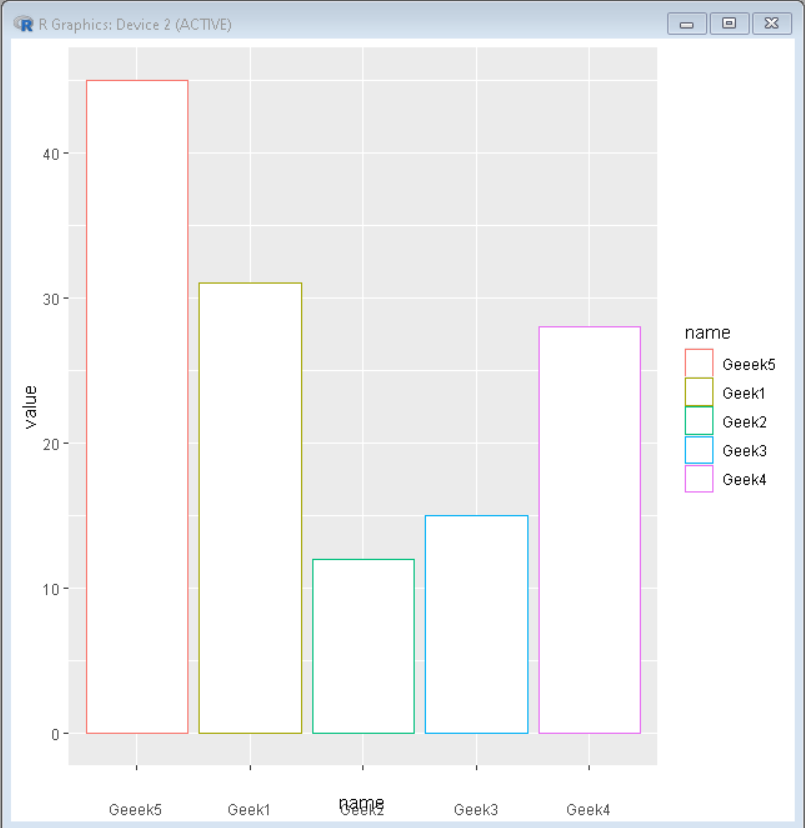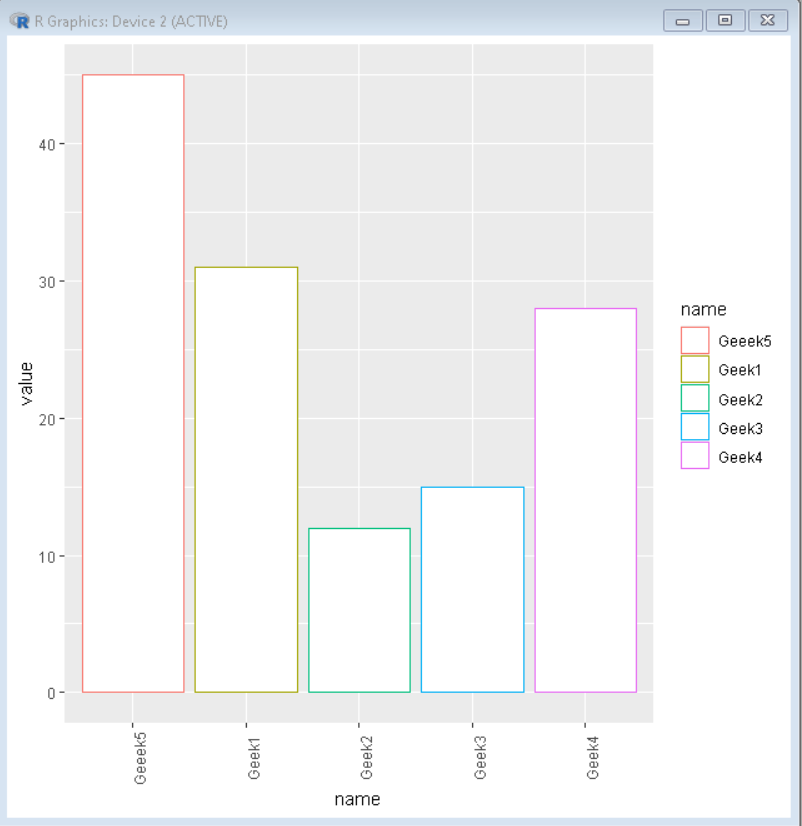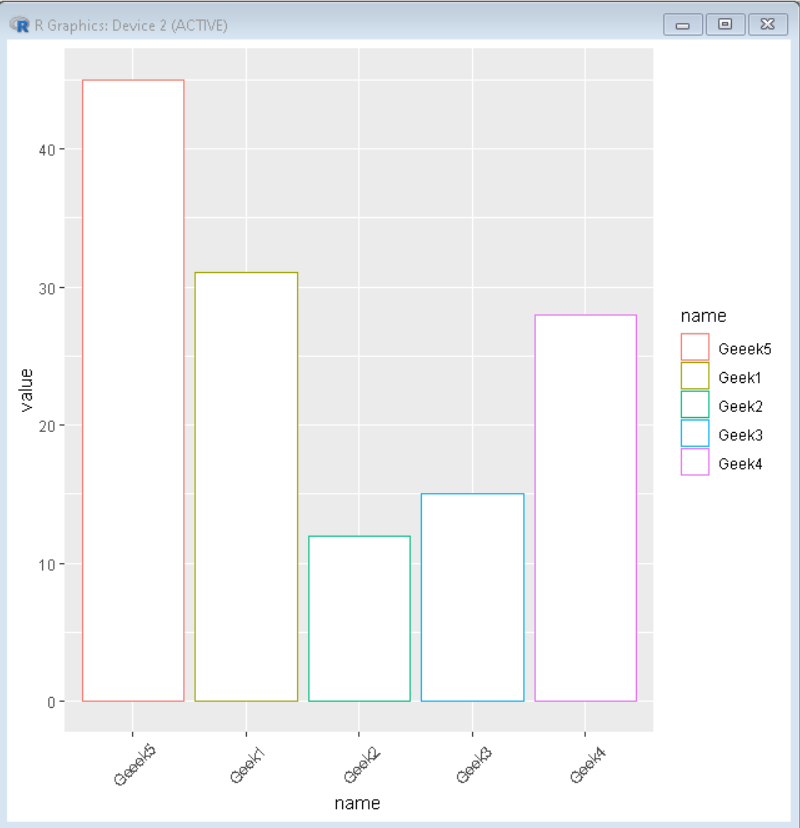Open In App

# Rotating and spacing axis labels in ggplot2 in R

In this article, we will discuss how to Rotate and space axis labels in the ggplot2 in the R Programming Language.

## Spacing the axis labels:

We can increase or decrease the space between the axis label and axis using the theme function. The axis.txt.x / axis.text.y parameter of theme() function is used to adjust the spacing using hjust and vjust argument of the element_text() function.

Syntax:

`plot + theme( axis.text.x / axis.text.y = element_text( hjust, vjust )`

where,

• hjust: determines the horizontal justification
• vjust: determines the vertical justification

Example:

In this example, we have added vertical space of 10 points using vjust command of theme function in the ggplot2 plot in the R Language.

## R

 `# Create sample data``set.seed``(5642)                            ``sample_data <- ``data.frame``(name = ``c``(``"Geek1"``,``"Geek2"``,``                                   ``"Geek3"``,``"Geek4"``,``                                   ``"Geeek5"``) ,``                          ``value = ``c``(31,12,15,28,45))` `# Load ggplot2 package``library``(``"ggplot2"``)` `# Create bar plot using ggplot() function``ggplot``(sample_data,``             ``aes``(name,value,, color=name)) +` `# geom_bar function is used to plot bars``# of barplot``geom_bar``(stat = ``"identity"``, fill=``"white"``)+` `# vjust is used to justify the label vertically``theme``(axis.text.x = ``element_text``(vjust=-10))`

Output:## Rotating Axis Labels

We can rotate the axis label and axis using the theme function. The axis.txt.x / axis.text.y parameter of theme() function is used to adjust the rotation of labels using the angle argument of the element_text() function.

Syntax:

`plot + theme( axis.text.x / axis.text.y = element_text( angle )`

where,

angle: determines the angle of rotation

Example:

In this example, we have made the rotation angle 90 degrees using the angle command of the theme function in the ggplot2 plot in the R Language. This makes the axis labels vertical.

## R

 `# Create sample data``set.seed``(5642)                            ``sample_data <- ``data.frame``(name = ``c``(``"Geek1"``,``"Geek2"``,``                                   ``"Geek3"``,``"Geek4"``,``                                   ``"Geeek5"``) ,``                          ``value = ``c``(31,12,15,28,45))` `# Load ggplot2 package``library``(``"ggplot2"``)` `# Create bar plot using ggplot() function``ggplot``(sample_data,``             ``aes``(name,value,, color=name)) +` `# geom_bar function is used to plot bars``# of barplot``geom_bar``(stat = ``"identity"``, fill=``"white"``)+` `# rotate axis label using axis.text.x parameter``# of theme() 90 degree rotation makes label``# vertical``theme``(axis.text.x = ``element_text``(angle = 90))`

Output:Example:

In this example, we have rotated the axis label by 45 degrees that made it overlapping with the plot. so we moved it down using the vjust parameter to avoid overlapping.

## R

 `# Create sample data``set.seed``(5642)                            ``sample_data <- ``data.frame``(name = ``c``(``"Geek1"``,``"Geek2"``,``                                   ``"Geek3"``,``"Geek4"``,``                                   ``"Geeek5"``) ,``                          ``value = ``c``(31,12,15,28,45))` `# Load ggplot2 package``library``(``"ggplot2"``)` `# Create bar plot using ggplot() function``ggplot``(sample_data,``             ``aes``(name,value,, color=name)) +` `# geom_bar function is used to plot bars``# of barplot``geom_bar``(stat = ``"identity"``, fill=``"white"``)+` `# rotate axis label using axis.text.x parameter of theme()``# vjust is used to justify the label to avoid``# overlapping with plot``theme``(axis.text.x = ``element_text``(angle = 45, vjust=0.5))`

Output: